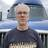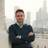Time Stamps for Status Changes

I have multiple "process status" fields that I want to time stamp when the status changes (or the last time it was changed).

I'm using both Yes/No and Choice type fields.

So when a status changes to Yes, I want to display the time that happens.

I tried using:

if 'Accepted By Processing' then
now()
end

Obviously the problem here is that this field is always changing to the Now time.  How do I lock in the time when a status field changes.

I've looked through the forum and could not find a formula for this.I assume you have Date/Time fields setup to accept the time stamp? If so, you can add something like this to your Yes/No and Choice fields...

`let t := this;`
`if t.'Yes / No' then`
`t.('Date / Time' := now())`
`else`
`t.('Date / Time' := null)`
`end`

`let t := this;`
`if text(t.Choice) = "YourValue" then`
`t.('Date / Time' := now())`
`else`
`t.('Date / Time' := null)`
`end`The code would go in the "Trigger after update" for the Yes/No and Choice fields.Sean,

Thank you for your response.  It looks like that sorts out my problem!  Big Thanks!!

Cheers!